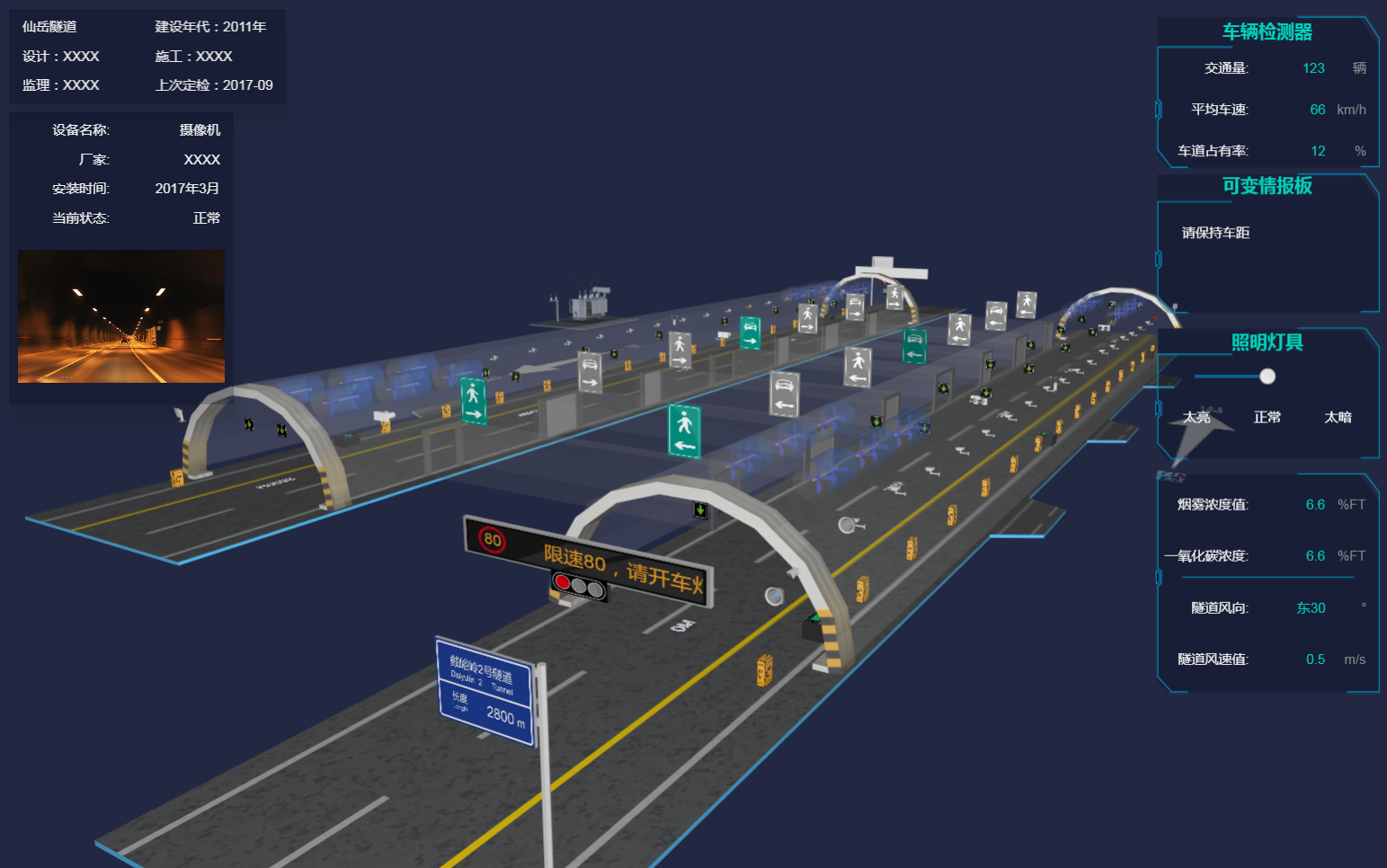2018-03-26 07:53:21

效果图代码实现

场景搭建

dm = new ht.DataModel();//数据容器 g3d = new ht.graph3d.Graph3dView(dm);// 3d 场景 g3d.addToDOM();//将场景添加到 body 中

addToDOM = function(){ var self = this,
view = self.getView(),//获取组件的底层 div style = view.style;
document.body.appendChild(view);//将组件底层div添加进body中 style.left = '0';//ht 默认将所有的组件的position都设置为absolute绝对定位 style.right = '0';
style.top = '0';
style.bottom = '0';
window.addEventListener('resize', function () { self.iv(); }, false);//窗口大小改变事件，调用刷新函数 }

JSON 反序列化

ht.Default.xhrLoad('./scenes/隧道1.json', function(text) {//xhrLoad 函数是一个异步加载文件的函数 var json = ht.Default.parse(text);//将 json 文件中的文本转为我们需要的 json 格式的内容 dm.deserialize(json);//反序列化数据容器，解析用于生成对应的Data对象并添加到数据容器 这里相当于把 json 文件中生成的 ht.Node 节点反序列化到数据容器中，这样数据容器中就有这个节点了 });

dm.each(function(data) { if (data.s('front.image') === 'assets/sos电话.png'){//对“电话”进行批量 data.s('batch', 'sosBatch');
} else if (data.s('all.color') === 'rgba(222,222,222,0.18)') {//逃生通道批量（透明度也会影响性能） data.s('batch', 'emergencyBatch');
} else if (data.s('shape3d') === 'models/隧道/摄像头.json' || data.s('shape3d') === 'models/隧道/横洞.json' || data.s('shape3d') === 'models/隧道/卷帘门.json') { if(!data.s('shape3d.blend'))//个别摄像头染色了 不做批量 data.s('batch', 'basicBatch');//基础批量什么也不做  } else if (data.s('shape3d') === 'models/大型变压器/变压器.json') {
data.s('batch', 'tileBatch');
data.setToolTip('单击漫游，双击车祸地点出现图标');
} else if (data.getDisplayName() === '地面') {
data.s('3d.selectable', false);//设置隧道“地面”不可选中  } else if (data.s('shape3d') === 'models/隧道/排风.json') {
data.s('batch', 'fanBatch');//排风扇的模型比较复杂，所以做批量  } else if (data.getDisplayName() === 'arrow') {//隧道两旁的箭头路标 if (data.getTag() === 'arrowLeft') data.s('shape3d.image', 'displays/abc.png'); else data.s('shape3d.image', 'displays/abc2.png');
data.s({ 'shape3d': 'billboard', 'shape3d.image.cache': true,//缓存，设置了 cache 的代价是需要设置 invalidateShape3dCachedImage 'shape3d.transparent': true //设置这个值，图片上的锯齿就不会太明显了（若图片类型为 json，则设置 shape3d.dynamic.transparent)  });
g3d.invalidateShape3dCachedImage(data);
} else if (data.getTag() === 'board' || data.getTag() === 'board1') {//隧道入口处的情报板 data.a('textRect', [0, 2, 244, 46]); //业务属性，用来控制文本的位置[x,y,width,height] data.a('limitText', '限速80，请开车灯');//业务属性，设置文本内容 var min = -245; var name = 'board' + data.getId();
window[name] = setInterval(function() {
circleFunc(data, window[name], min)//设置情报板中的文字向左滚动，并且当文字全部显示时重复闪烁三次 }, 100);
} //给逃生通道上方的指示板 动态设置颜色 var infos = ['人行横洞1', '人行横洞2', '人行横洞3', '人行横洞4', '车行横洞1', '车行横洞2', '车行横洞3'];
infos.forEach(function(info) { if(data.getDisplayName() === info) {
data.a('emergencyColor', 'rgb(138, 138, 138)');
}
});

infos = ['车道指示器', '车道指示器1', '车道指示器2', '车道指示器3'];
infos.forEach(function(info) { if (data.getDisplayName() === info) {
createBillboard(data, 'assets/车道信号-过.png', 'assets/车道信号-过.png', info)//考虑到性能问题 将六面体变换为 billboard 类型元素  }
});
});

逻辑代码

情报板滚动条

function circleFunc(data, timer, min) {//设置情报板中的文字向左滚动，并且当文字全部显示时重复闪烁三次 var text = data.a('limitText');//获取当前业务属性 limitText 的内容 data.a('textRect', [data.a('textRect')-5, 2, 244, 46]); //设置业务属性 textRect 文本框的坐标和大小 if (parseInt(data.a('textRect')) <= parseInt(min)) {
data.a('textRect', [255, 2, 244, 46]);
} else if (data.a('textRect') === 0) {
clearInterval(timer); var index = 0; var testName = 'testTimer' + data.getId();//设置多个 timer 是因为能够进入这个函数中的不止一个 data，如果在同一时间多个 data 设置同一个 timer，那肯定只会对最后一个节点进行动画。后面还有很多这种陷阱，要注意 window[testName] = setInterval(function() {
index++; if(data.a('limitText') === '') {//如果情报板中文本内容为空 setTimeout(function() {
data.a('limitText', text);//设置为传入的 text 值 }, 100);
} else {
setTimeout(function() {
data.a('limitText', ''); //若情报板中的文本内容不为空，则设置为空 }, 100);
} if(index === 11) { //重复三次   clearInterval(window[testName]);
data.a('limitText', text);
}
}, 100);

setTimeout(function() {
timer = setInterval(function() {
circleFunc(data, timer, min) //回调函数 }, 100);
}, 1500);
}
}node.s({ 'shape3d': 'billboard', 'shape3d.image': imageUrl, 'shape3d.image.cache': true });
g3d.invalidateShape3dCachedImage(node); //还记得用 shape3d.image.cache 的代价么？

Camera 缓慢偏移

function setCenter(center, finish) {//设置“目标”位置 var c = g3d.getCenter().slice(0), //获取当前“目标”位置，为一个数组，而 getCenter 数组会在视线移动的过程中不断变化，所以我们先拷贝一份 dx = center - c, //当前x轴位置和目标位置的差值 dy = center - c,
dz = center - c; // 启动 500 毫秒的动画过度  ht.Default.startAnim({
duration: 500,
action: function(v, t) {
g3d.setCenter([ //将“目标”位置缓慢从当前位置移动到设置的位置处 c + dx * v,
c + dy * v,
c + dz * v
]);
}
});
}; function setEye(eye, finish) {//设置“眼睛”位置 var e = g3d.getEye().slice(0),//获取当前“眼睛”位置，为一个数组，而 getEye 数组会在视线移动的过程中不断变化，所以我们先拷贝一份 dx = eye - e,
dy = eye - e,
dz = eye - e; // 启动 500 毫秒的动画过度  ht.Default.startAnim({
duration: 500,
action: function(v, t) {//将 Camera 位置缓慢地从当前位置移动到设置的位置  g3d.setEye([
e + dx * v,
e + dy * v,
e + dz * v
]);
}
});
};

var p3 = e.data.p3(), //获取事件对象的三维坐标 s3 = e.data.s3(),//获取事件对象的三维尺寸 r3 = e.data.r3();//获取事件对象的三维旋转值  setCenter(p3);//设置“目标”位置为当前事件对象的三维坐标值 if (r3 !== 0) {//如果节点的 y 轴旋转值 不为 0 if (parseFloat(r3.toFixed(5)) === parseFloat(-3.14159)) { //浮点负数得做转换才能进行比值 setEye([p3, p3+s3, p3 * Math.abs(r3*2.3/6)]);//设置camera 的目标位置  } else if (parseFloat(r3.toFixed(4)) === parseFloat(-1.5708)) {
setEye([p3 * Math.abs(r3/1.8), p3+s3, p3]);  } else {
setEye([p3 *r3, p3+s3, p3]);
}
} else {
setEye([p3, p3+s3*2, p3+1000]);
}

事故模拟现场

g3d.addInteractorListener(function(e) { if(e.kind === 'doubleClickData') { if (e.data.getTag() === 'jam') return;//有“事故”图标节点存在 if (e.data.s('shape3d') === 'models/大型变压器/变压器.json') {//如果双击对象是变压器 index++; var jam = dm.getDataByTag('jam');//通过唯一标识tag标签获取“事故”图标节点对象 if(index === 1){ var jam = dm.getDataByTag('jam');
jam.s({ '3d.visible': true,//设置节点在 3d 上可见 'shape3d': 'billboard',//设置节点为 billboard 类型 'shape3d.image': 'assets/车祸.png', //设置 billboard 的显示图片 'shape3d.image.cache': true,//设置 billboard 图片是否缓存 'shape3d.autorotate': true,//是否始终面向镜头 'shape3d.fixSizeOnScreen': [30, 30],//默认保持图片原本大小，设置为数组模式则可以设置图片显示在界面上的大小  });
g3d.invalidateShape3dCachedImage(jam);//cache 的代价是节点需要设置这个函数  } else {
jam.s({ '3d.visible': false//第二次双击变压器就将所有一切恢复“事故”之前的状态  });
dm.each(function(data) { var p3 = data.p3(); if ((p3 < jam.p3()) && data.getDisplayName() === '车道指示器1') {
data.s('shape3d.image', 'assets/车道信号-过.png');
} if(data.getTag() === 'board1') {
data.a('limitText', '限速80，请开车灯');
}
});
index = 0;
}

}
}
});

else if (e.kind === 'clickData'){//点击图元 timer = setTimeout(function() {
clearTimeout(timer); if (e.data.getTag() === 'jam') {//如果是“事故”图标节点 createDialog(e.data);//创建一个对话框  }
}, 200);
}function createForm4(node, dialog) {//弹出框右边的表单 var form = new ht.widget.FormPane();//表单组件 form.setWidth(200);//设置表单组件的宽 form.setHeight(200);//设置表单组件的高 var view = form.getView();//获取表单组件的底层 div  document.body.appendChild(view);//将表单组件添加到 body 中 var infos = [ '编辑框内容为：2辆', '编辑框内容为：客车-客车', '编辑框内容为：无起火', '编辑框内容为：超车道' ];
infos.forEach(function(info) {
], [0.1]);//第二个参数为行宽度，小于1的值为相对值  });

{
button: {//添加一行的“确认”按钮 label: '确认',
onClicked: function() {//按钮点击事件触发 dialog.hide();//隐藏对话框 dm.each(function(data) { var p3 = data.p3(); if ((p3 < node.p3()) && data.getDisplayName() === '车道指示器1') {//改变“车道指示器”的显示图片为红色×，这里我是根据“事故”图标节点的坐标来判断“车道显示器”是在前还是在后的 data.s('shape3d.image', 'assets/车道信号-禁止.png');
} if(data.getTag() === 'board1') {//将隧道口的情报板上的文字替换 data.a('limitText', '超车道两车追尾，请减速慢行');
}
});
}
}
}
], [0.1]); return form;
}